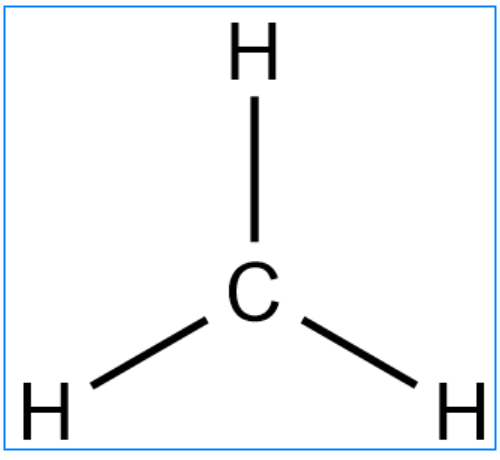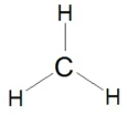# Problem: Calculate the formal charge on carbon.

###### FREE Expert Solution

We’re being asked to determine the formal charge of carbon in the given Lewis structure. Recall that the formula for the formal charge is:

The given Lewis structure is:86% (114 ratings)###### Problem Details

Calculate the formal charge on carbon.